Area and Perimeter of - Circle, Parallelogram, Square, Rectangle

Chapter 9 Class 8 Mensuration
Serial order wise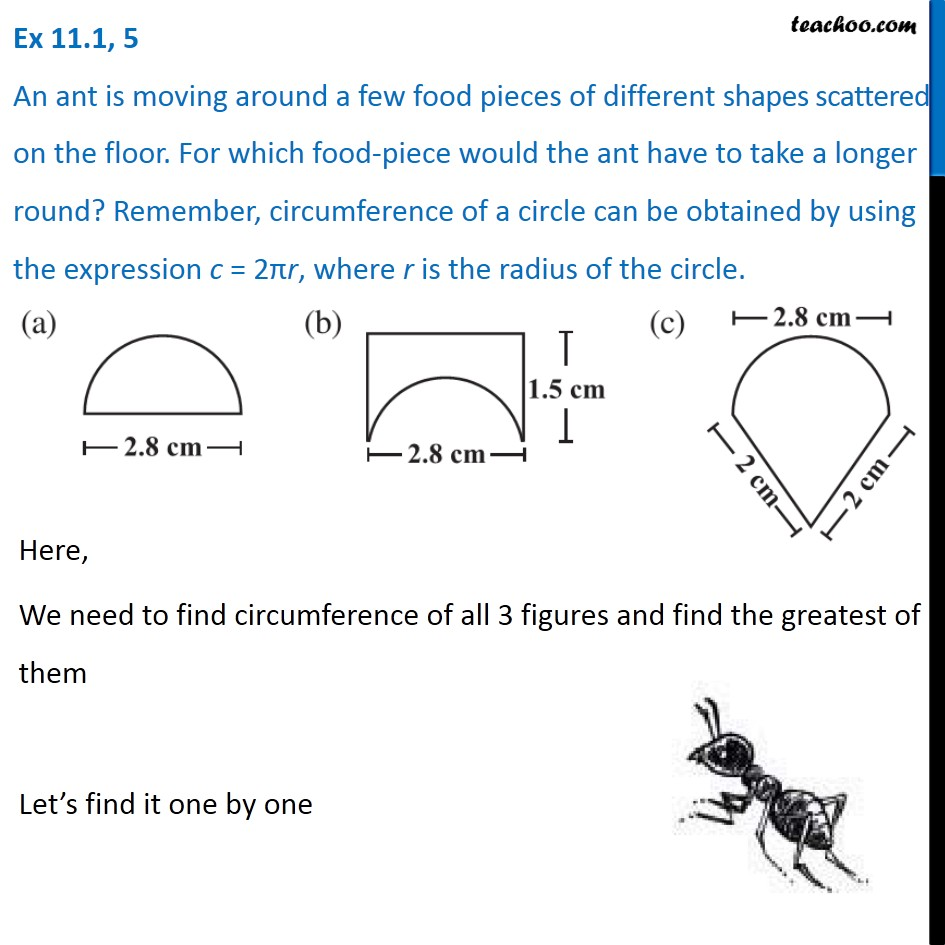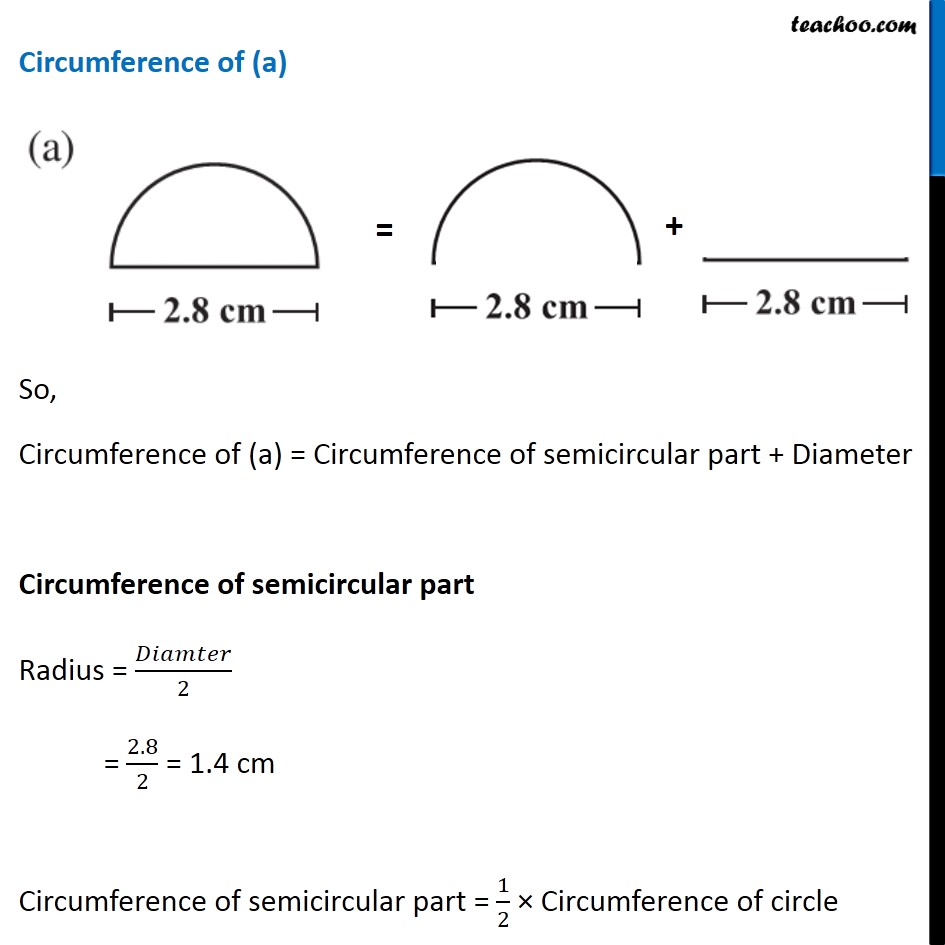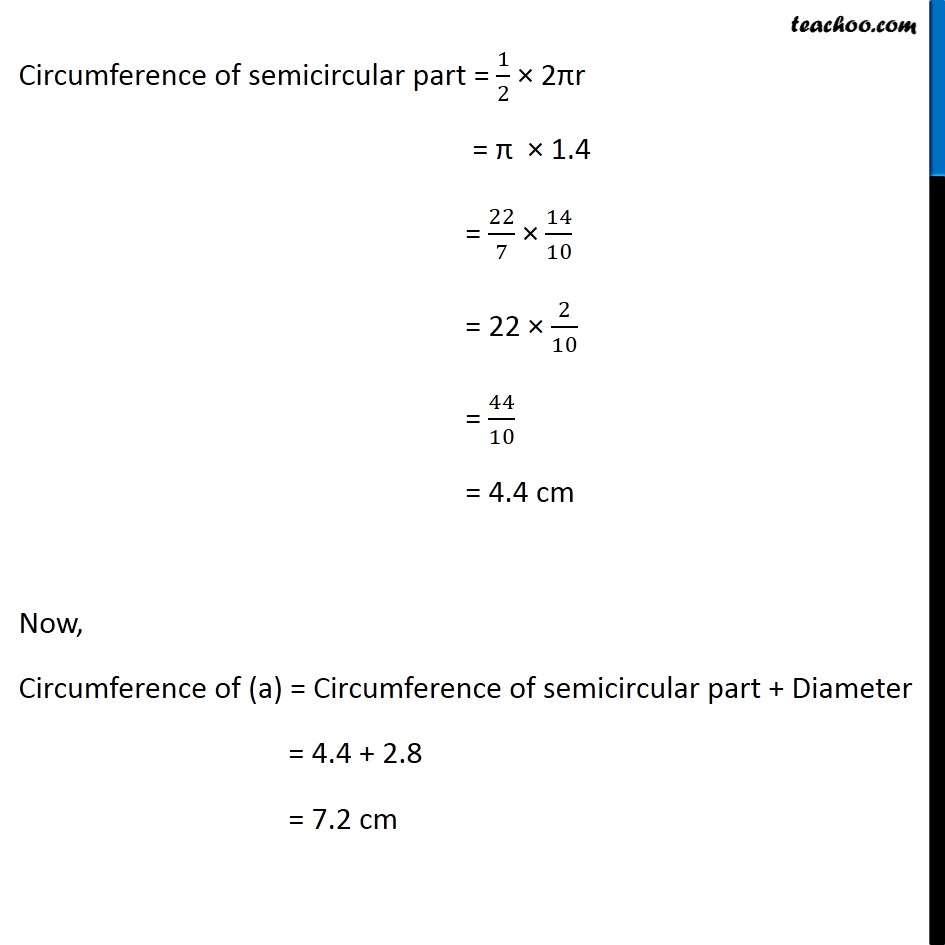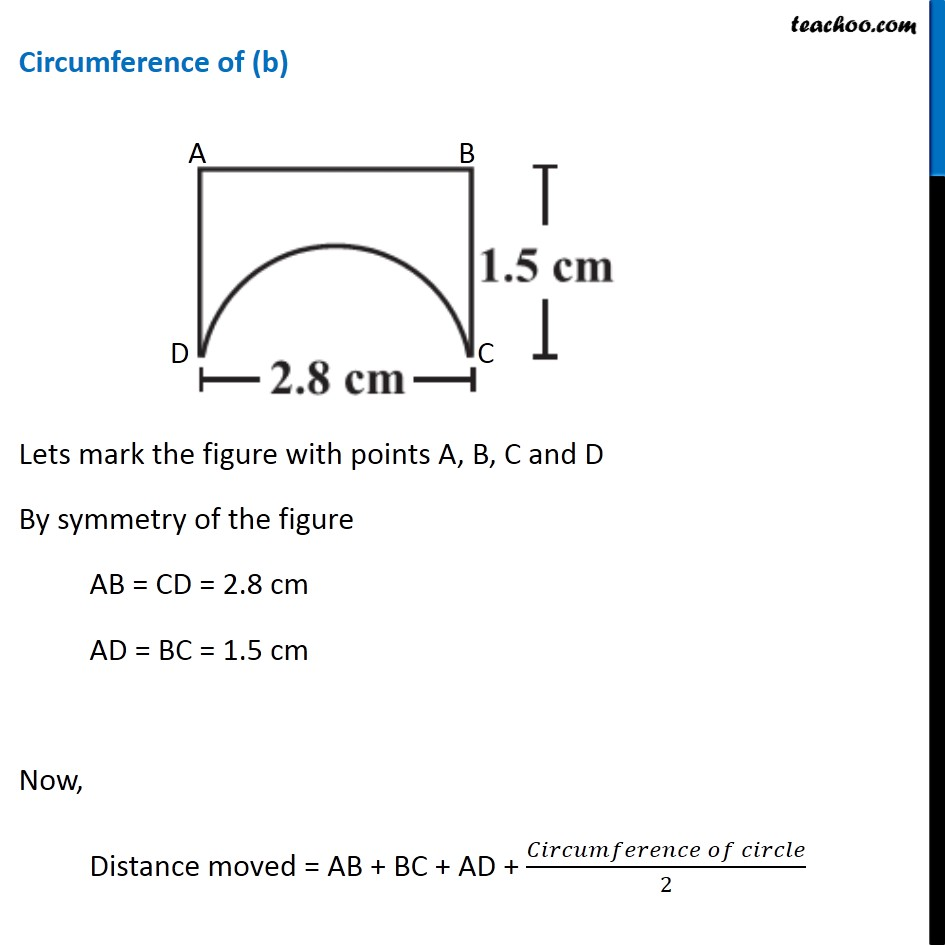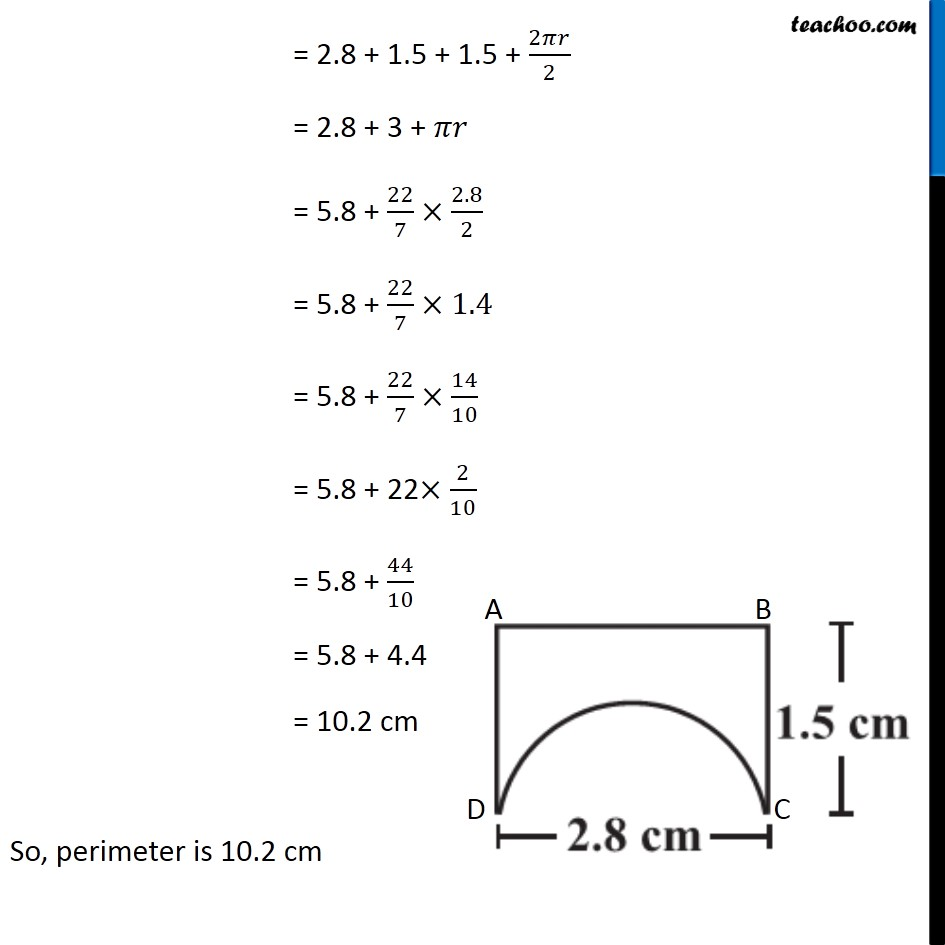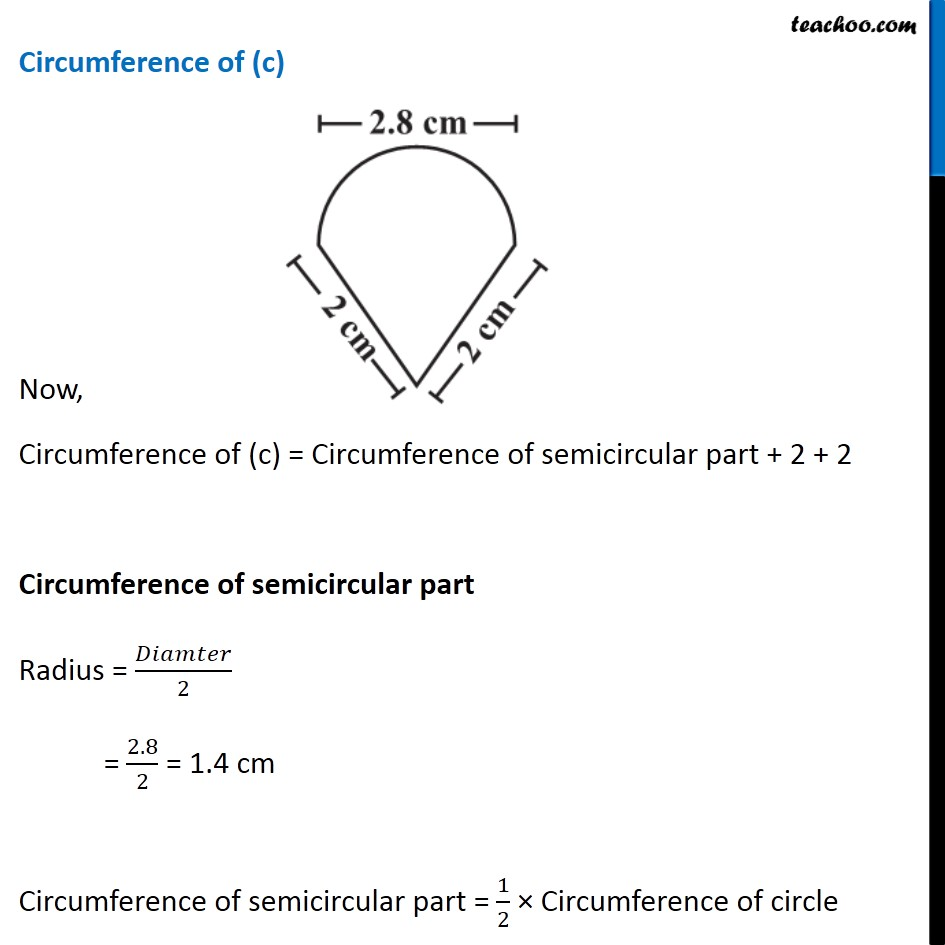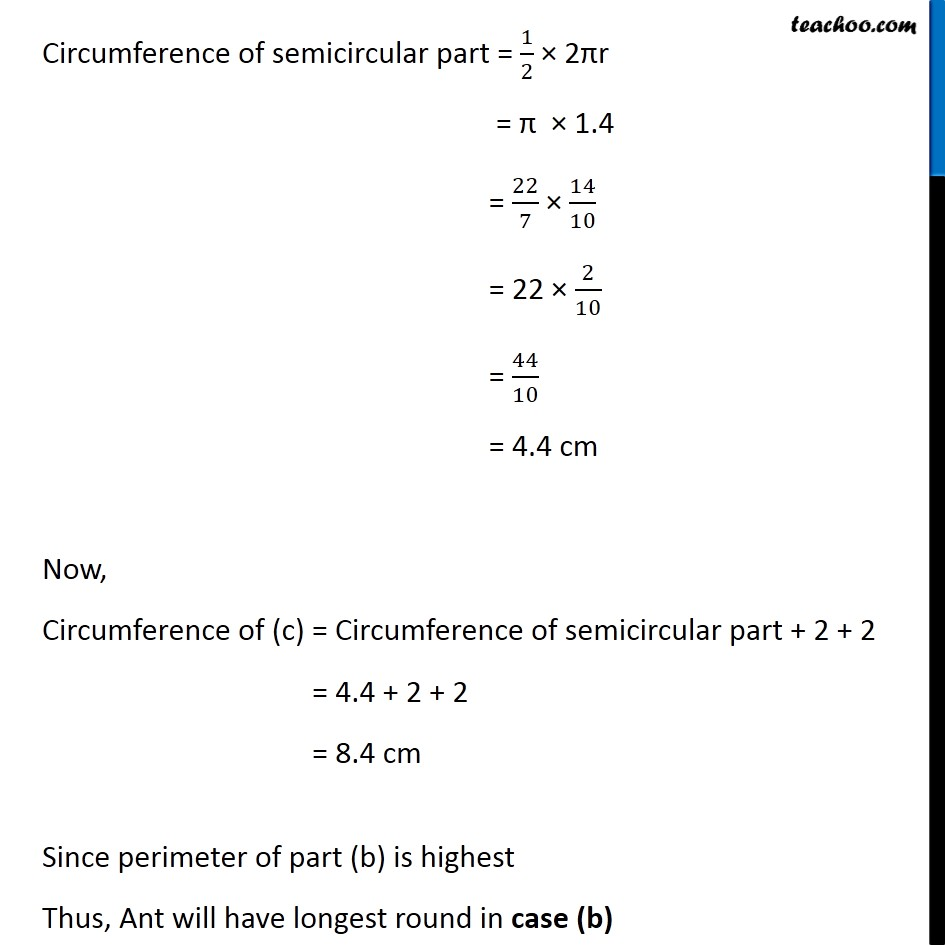Learn in your speed, with individual attention - Teachoo Maths 1-on-1 Class

### Transcript

Question 5 An ant is moving around a few food pieces of different shapes scattered on the floor. For which food-piece would the ant have to take a longer round? Remember, circumference of a circle can be obtained by using the expression c = 2πr, where r is the radius of the circle. Here, We need to find circumference of all 3 figures and find the greatest of them Let’s find it one by one Circumference of (a) So, Circumference of (a) = Circumference of semicircular part + Diameter Circumference of semicircular part Radius = 𝐷𝑖𝑎𝑚𝑡𝑒𝑟/2 = 2.8/2 = 1.4 cm Circumference of semicircular part = 1/2 × Circumference of circle Circumference of semicircular part = 1/2 × 2πr = π × 1.4 = 22/7 × 14/10 = 22 × 2/10 = 44/10 = 4.4 cm Now, Circumference of (a) = Circumference of semicircular part + Diameter = 4.4 + 2.8 = 7.2 cm Circumference of (b) Lets mark the figure with points A, B, C and D By symmetry of the figure AB = CD = 2.8 cm AD = BC = 1.5 cm Now, Distance moved = AB + BC + AD + (𝐶𝑖𝑟𝑐𝑢𝑚𝑓𝑒𝑟𝑒𝑛𝑐𝑒 𝑜𝑓 𝑐𝑖𝑟𝑐𝑙𝑒)/2 = 2.8 + 1.5 + 1.5 + 2𝜋𝑟/2 = 2.8 + 3 + 𝜋𝑟 = 5.8 + 22/7×2.8/2 = 5.8 + 22/7×1.4 = 5.8 + 22/7×14/10 = 5.8 + 22×2/10 = 5.8 + 44/10 = 5.8 + 4.4 = 10.2 cm So, perimeter is 10.2 cm Circumference of (c) Now, Circumference of (c) = Circumference of semicircular part + 2 + 2 Circumference of semicircular part Radius = 𝐷𝑖𝑎𝑚𝑡𝑒𝑟/2 = 2.8/2 = 1.4 cm Circumference of semicircular part = 1/2 × Circumference of circle Circumference of semicircular part = 1/2 × 2πr = π × 1.4 = 22/7 × 14/10 = 22 × 2/10 = 44/10 = 4.4 cm Now, Circumference of (c) = Circumference of semicircular part + 2 + 2 = 4.4 + 2 + 2 = 8.4 cm Since perimeter of part (b) is highest Thus, Ant will have longest round in case (b)